• matlab：char2cell

2014-10-23 10:38:06matlab
• matlab中double、char和cell的互转

万次阅读 多人点赞 2017-12-23 17:20:18
哎，对会的人来说可能真的是一个函数的事，但是对于我这种对matlab不熟的初学者，真的不是那么简单，其中涉及各种转换，比如double，charcell的互。 比如我有一个txt文档是这样子的： 我要的信息是该txt文档...

小鱼这两天被导师嫌弃，说一个txt文档转换为mat格式都花了半天时间，不就一个save函数的事吗。哎，对会的人来说可能真的是一个函数的事，但是对于我这种对matlab不熟的初学者，真的不是那么简单，其中涉及各种转换，比如double，char，cell的互转。
比如我有一个txt文档是这样子的：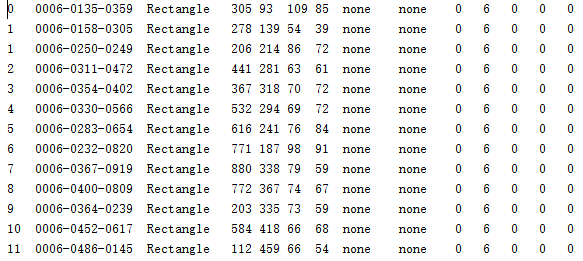我要的信息是该txt文档的第4-7这四列
首先我们要读入txt文档，读入txt文档的函数为：A=importdata（filename.txt），读入之后才可以对文档进行处理，读入之后一般会有data和textdata两个字段，你需要那个就直接A.data或A.textdata获取。
获取完之后就存在这转换问题了，比如我们最终要的格式为：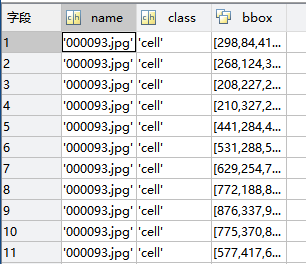可以看出有些事char字符型，有的是矩阵型
所以接下来就是转换问题，前提是要弄清楚你要的是什么类型，有的是什么类型
1、cell2mat：将cell转换为mat的char型
2、str2num：将mat从char转换为double型
3、cellstr：将char转cell
4、num2str：将double转char
5、num2cell：将double直接转cell

比如：

files = dir( ['\*.txt']);
name = files.name;
A = importdata(name);
info = A.textdata;
B(1).objects(1).bbox(:,1)=str2num(cell2mat(info(j,4)));         B(1).objects(1).bbox(:,2)=str2num(cell2mat(info(j,5)));

就可以获取txt文档中第4列和第5列的数据存入一个B的struct中。
这部分如果有什么问题需要小鱼解答的，可以留下评论，小鱼会的话一定帮忙解决哦

展开全文matlab
• MATLAB-char,str转cell类型

万次阅读 2018-12-17 14:45:38
MATLAB中我们的char数组一般都是不定长的。比如你乍一看：好像是1*1 但是实际上却是： 解决 一般是将其转化为1*1 cell类型。 核心操作： cellstr() 代码 newpath = cellstr(path); 此时 ...

综述

MATLAB中我们的char数组一般都是不定长的。比如你乍一看：好像是1*1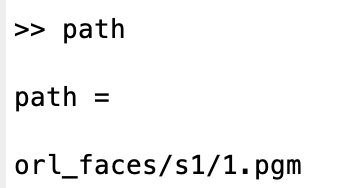但是实际上却是：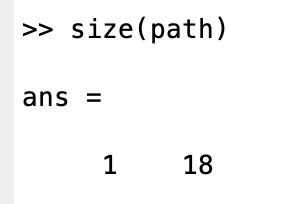解决

一般是将其转化为1*1 cell类型。
核心操作：

cellstr()

代码

newpath =  cellstr(path);

此时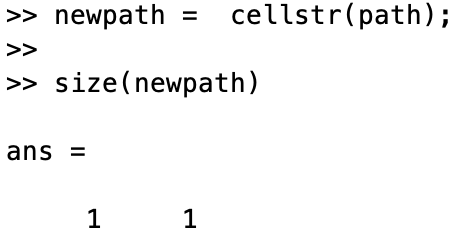展开全文matlab char数组 char str
• MATLAB - 将char转换为1x5单元格(MATLAB - Convert char to 1x5 cell)我有5个等级F，F，D，B，A等级= FFDBA。 我不知道如何将每个年级转换为单元格数组？ 即ans = [F] [F] [D] [B] [A]。 cellstr(grade)仅创建1x1...

MATLAB - 将char转换为1x5单元格(MATLAB - Convert char to 1x5 cell)

我有5个等级F，F，D，B，A等级= FFDBA。 我不知道如何将每个年级转换为单元格数组？ 即ans = [F] [F] [D] [B] [A]。 cellstr(grade)仅创建1x1单元阵列[FFDBA]。 我想要一个1x5的单元格。

I have 5 grades F, F, D, B, A in grade = FFDBA . I am not sure how to convert each grade to a cell array? i.e. ans= [F] [F] [D] [B] [A]. cellstr(grade) only creates a 1x1 cell array [FFDBA]. I'd like a 1x5 cell.

原文：https://stackoverflow.com/questions/39824138

更新时间：2020-02-04 01:46

最满意答案

当你想将数组拆分为相同大小的部分时，使用num2cell函数将数组转换为单元格。

ans =

'F' 'F' 'D' 'B' 'A'

Use the num2cell function to convert an array to a cell, when you want to split the array into same-size parts.

ans =

'F' 'F' 'D' 'B' 'A'

2016-10-03

相关问答

您可以将单个字符数组收集到单个字符数组中，然后使用str2num进行转换： labels_numeric = str2num([labels{:}]);

或者更简单，只需使用str2double ： labels_numeric = str2double(labels);

You can collect the individual character arrays into a single character array, then use str2num to convert it: l

...

在这种情况下，您无法真正信任函数disp 。 逻辑值将显示0或1 。 例如： >> disp(false)

0

你应该使用class函数测试x的数据类型，我相信你会看到它返回logical ： >> x = false;

>> class(x)

ans =

logical

如果你想强制它进行转换，你可以将logical转换为double精度，如下所示： y = char(double(x));

但是，您将只得到一个null或开头的标题字符( ASCII代码为0和1 )。 You c

...

因为你想知道每个21-char字符串使用了多少次： 1) sort the cell

2) count how many times each string is used in a for loop.

你的代码是O(n ^ 2)所以它很慢。 这应该不到一分钟。 根据您的代码 B=sort(A{2});

U=sort(unique(B));

C=zeros(numel(U),1);

cnt = 1;

for j=1:numel(B)

if strcmp(U(cnt),B(j))

...

您可以使用大括号( {} )从单元格中获取字符串： x='AGCT';

y(1) = {x};

y{1}

ans =

AGCT

您可以将索引操作符串在一起，以直接从单元格数组中获取单个字符。 例如： y{1}(2)

ans =

G

还要记住， char函数可以通过垂直连接字符串将字符串的单元格数组转换为2D字符数组，同时根据需要使用空格填充： S = char(C) ，当C是字符串的单元格数组时，将C每个元素放入字符数组S的行中。 使用CELLSTR转换回来。 这样你就可以将你的整

...

你几乎拥有它。 您需要垂直连接逗号分隔列表r{:} ，而不是水平连接。 所以，使用 cellstr(vertcat(r{:}))

You almost have it. You need to concatenate the comma-separeted list r{:} vertically, not horizontally. So, use cellstr(vertcat(r{:}))

您可以使用函数cellstr执行此操作，如Divakar所建议的那样。 A = ['hello'

'hillo'

'hello']

C = cellstr(A)

C =

'hello'

'hillo'

'hello'

You can use the function cellstr to do this, as proposed by Divakar. A = ['hello'

'hillo'

'hello']

C =

...

你创建了一个字符串： value = '0xaa';

4个字符， ['0' 'x' 'a' 'a'] 。 在MATLAB中，您通常不会逐位处理变量，但如果需要，请尝试： temp = int8(hex2dec('aa'));

You have created a string: value = '0xaa';

Of 4 characters, ['0' 'x' 'a' 'a']. In MATLAB you don't generally handle variables bit wise,

...

如果input_array是您的输入数组，请尝试此基于cellfun + num2str的方法 - cellfun(@(x) num2str(x,'%1d'), input_array,'uni',0)

示例 - %// Input array

input_array = {randi(9,1,5)-1;randi(9,1,2)-1}

%// Display the cell array values for verification of results later on

celldisp(

...

您可以使用textscan轻松地将此字符串解析为多个数字。 a = '-2.2748E+01,-2.3454E+00,5.2434E00';

data = textscan(a, '%f', 'delimiter', ',');

data = data{1};

% -22.7480

% -2.3454

% 5.2434

You can use textscan to easily parse this string out into multiple numbers. a = '

...

ans =

'F' 'F' 'D' 'B' 'A'

Use the num2cell function to convert an array to a cell, when you want to split the array into same-size parts. grade =

...

展开全文• 函数 S = cell2char(C) % % 将字符串元胞数组的内容转换为字符％ 大批。 单元格 C 的内容按元素读取，并且% 转换为长度为 MAXCOL 的字符数组，其中 MAXCOL 为% 数组最长字符串的长度。 % 因此得到的字符数组 S 的...matlab
• matlab实现cell转str（元胞数组字符）描述函数代码1输出1代码2输出2 描述 matlab实现cell转str（元胞数组字符） 函数 通过大括号实现 A = A{1} 代码1 A = {'wang',2,3}; B = A{1} C = A{2} D = A{3} 输出1 ...

描述

matlab实现cell转str、double（元胞数组转字符、数值）

函数

方法一

通过大括号{}实现，数组中存储的是什么格式，输出的就是啥格式。
A = A{1,1}
注意小括号（），仅仅只具有提取功能，输出还是元胞结构。
比如

A = {'1','wang';'2','yomglin'}
A{1,1}          % 提取第一行第一列的元胞里面的数据‘1’，输出‘double’格式，就是存储在元胞脸面数据的原格式
A{1,2}			% 提取第一行第二列的元胞里面的字符串‘wang’，输出‘char’格式，就是原格式
%------------------------------------
A(2,1)			% 注意！  换成圆括号之后，输出的均为元胞结构，仅仅只具有提取功能{‘2’}。
A(2,2)			% 提取元胞结构 {‘yonglin’}

方法二

函数 cell2mat
将元胞数组转换为基础数据类型的普通数组(详细见说明文档，链接如下)
https://ww2.mathworks.cn/help/matlab/ref/cell2mat.html?s_tid=doc_ta

A = {'1','wang';'2','yomglin'}
cell2mat(A(1,1))      % 将提取的第一行第一列的元胞结构的{‘1’}，转换成double数值类型1
cell2mat(A(1,2)) 		%同理

展开全文matlab
• 之所以会涉及使用cell，是因为涉及字符串、数字在同一个“矩阵”，这时候需要使用cell进行存储，在数据处理时候又需要进行cell转矩阵，因此掌握这些转换方法可以使得程序更加简洁高效。 使用到的语法 cell2mat % ...
• 零散MATLAB资料分享-matlabcellchar，字符串与字符数组.docx 最近在用MATLAB做一个项目，自然需要查很多东西，在网上搜集了一点资料，并非原创。本着分享的精神，希望能帮助到部分人。 包括以下内容 ...matlab
• 句法： cellmat = char2cell(s,delim,rowseparate,trim); cellmat = char2cell(s,delim,rowseparate); cellmat = char2cell(s,delim); cellmat = char2cell(s); 如果“s”是字符数组，则可以根据分隔符（可以是...matlab
• MATLAB数据转化num,str,cell,char

万次阅读 2019-05-26 15:12:28
数字变为字符串 a=num2str(123) cell2mat：将cell转换为mat的char型 str2num：将mat从char转换为double型 cellstr：将char转cell num2str：将double转char num2cell：将double直接转cell ...
• 我想把cell变成char型，然后就自然地想到cell2str，遗憾的是matlab中没有这个函数。就像没有str2cell一样。实现str2cell功能的函数是cellstr，将字符数组转换为cell型字符串数组函数语法为c=cellstr(S)它的作用是：...
• str2num： 将字符数组或字符串转换为数值数组 cell2mat： 将元胞数组转换为基础数据类型的普通数组matlab
• matlab开发-cell2char。函数将字符串的单元格数组转换为字符数组。
• matlab中char函数 30天的30个Excel函数：08 – CHAR (30 Excel Functions in 30 Days: 08 – CHAR) Yesterday, in the 30XL30D challenge, we found character code numbers with the CODE function, and used it ...
• Cell Array的定义：顾名思义，Cell Array是由Cell构成的Array。Cell可以包含各种数据类型。可以使用()取cell array的子集，使用{}取cell array指定位置的元素值。 比如：myCell = {1, 2, 3;'text', rand(5,10,2), {...
• matlab中如何将cell数组转化成数值型矩阵

万次阅读 多人点赞 2018-09-12 17:07:06
将cell数组转化成矩阵 1.若cell中的元素为数值型，则使用cell2mat...2. 若cell中的元素为含有数字的字符串，则使用 str2num 和 char 函数。 如：    注：1）当cell中的元素为含有数字的字符串时，使用cell2...Matlab
• cell转换为char类型，matlab中提供了cell2mat函数，可以将cell类型转换为char类型，但会破坏原来cell的结构，使原来多维数组变为一维，在使用的时候有需要额外拆分，相当麻烦，如下图所示。 在string转换cell...matlab
• num2cell函数是将数组转换为相同大小的元胞数组。 一.语法 C =num2cell(A) C =num2cell(A,dim) 1.输入参数 A-输入 任何类型的多维数组 输入，指定为任何类型的多维数组。 数据类型：double|single|int8...matlab
• MATLAB 的数据类型与其它语言类似，下文将对MATLAB中的数据类型做一总结注意：在matlab中有whos()函数和class()函数等可以查看变量数据类型，使用格式如下代码>> class(a) %%注意此处使用class的时候必须要...
• 转载自：...我想把cell变成char型，然后就自然地想到cell2str，遗憾的是matlab中没有这个函数。就像没有str2cell一样。实现str2cell功能的函数是cellstr，将字符数组转换为ce...
• 夏恩觉得在 MATLAB 内的数据型态，最好用的就是细胞数组。最头疼的呢，也是细胞数组。就如同 python 的 List 型态，我们可以放心地放入任何数据，不会受到任何制裁。好用的前提是，我们得先明白在不同数据格式间的...
• 先上代码，这里我要读表格数据，结果读出来之后发现是cell，没有办法直接画图，先得进行数据转化，过程如下。 close all;clear all;clc [num,txt,raw]=xlsread('cupfile20210329093017302-sig',...先把cell转变成charmatlab 可视化
• matlabcell数据转换

千次阅读 2018-09-21 15:33:54
由于经常从txt文件读取数据，选用textscan函数读取txt文件。读取的数据经常是cell形式的，经常需要转换为mat形式，网上学了两种方式，记录一下： 1.strs=cellfun(@str2num, cell); 2.str=char(cell); data=char2...
• Matlab中cell类型转成str类型

千次阅读 2018-03-01 11:27:00
在利用xlsread函数的时候，raw和txt图均为Cell类型，要进行转换为Str类型。用char这个函数就可以了：>> cscs = 'abc' 'defg' 'hi'>> s = char( cs )s =abc defghi >> whos Name Size Bytes Class ......

matlab中char转cellmatlab 订阅